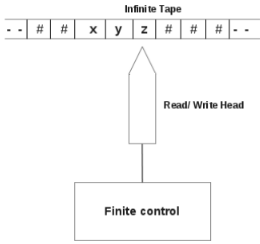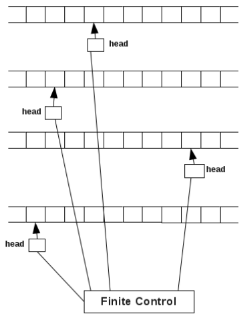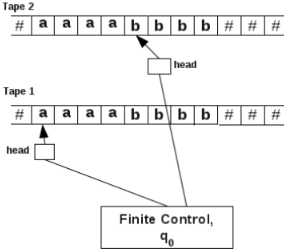Courses

Types of TM: Turing Machines Notes | Study Theory of Computation - Computer Science Engineering (CSE)

Computer Science Engineering (CSE): Types of TM: Turing Machines Notes | Study Theory of Computation - Computer Science Engineering (CSE)

The document Types of TM: Turing Machines Notes | Study Theory of Computation - Computer Science Engineering (CSE) is a part of the Computer Science Engineering (CSE) Course Theory of Computation.
All you need of Computer Science Engineering (CSE) at this link: Computer Science Engineering (CSE)

Part IV. Types of TM

5 Two Way Infinite Turing Machines

The TM we discussed so far had a tape that was finite on the left end and infinite on the right end.
Two way infinite TM is an extension to this such that tape is infinite at both ends. This is shown below:Thus on both sides of the tape, there is an infinite sequence of blank symbols (#).
This model does not provide any additional computational capability.

6 Multitape Turing Machines
A multitape TM consists of multiple tapes. Each tape has a separate head.
This is shown below:There are n tapes, each divided into cells. The first tape holds the input string. Initially, all the other tapes hold the blank
symbol, #.
A head has three possible options:
to move towards left (L),
to move towards right (R), or
to remain stationary (N).
All the heads are connected to a finite control. Finite control is in a state at an instant.
1. Initially, finite control is in state q0.
2. Head in the lowermost tape points to the cell containing leftmost symbol of the input string.
3. All the cells in the upper tapes contain # symbol.

When a transition occurs,
1. Finite control may change its state.
2. Head reads the symbol from the current cell and writes a symbol on it.
3. Each head can move towards left (L), right (R) or stay stationary (N).

An example transition function for a 4 tape TM is given below:
δ(q1, [a1, a2, a3, a4]) = (q2, [b1, b2, b3, b4], [L, R, R, N])
Here the finite control is in state q1, It reads the symbol a1from the uppermost tape, a2from the next uppermost tape and so on.
After reading, finite control changes its state to q2, and replaces the symbol a1by b1, a2by b2, a3by b3, a4by b4.
After this head in the upper most tape turns left. Head in the 2nd tape turns right. Head in the 3rd tape turns right. Head in the 4th tape remains stationary.
The advantage of using multi tape TM can be seen from the following example.
Example:
Consider the language L = {anbn|n ≥ 1}.
In a normal TM, head has to move back and forth to match each pair of symbols a and b. On a multitape TM, no such movements are needed.
This is done by making a copy of the input string in another tape.

Let the input string be aaaabbbb.
Let the input srtring be in tape 1. Make a copy of this string in tape 2.The head of tape 1 is positioned on the first a of the input string. Head of tape 2 is positioned on the first b of the input string.
Now the heads advance on both tapes simultaneously towards right and the string is accepted if there are equal number of a’s and b’s in the string.
This will happen if the head on tape 1 encounters the first b and the head on tape 2 encounters the first # simultaneously.

7 Universal Turing Machines
In the previous sections, a separate TM was designed for each language.
For example, for the language, L = {anbn|n ≥ 1}, we designed a TM.
For the language, L = {anbncn|n|n ≥ 1}, we designed another TM and so on.
A Universal turing Machine (UTM) takes the code of a normal TM and a string w, and checks whether w is recognised by TM.
This is done as follows:
Consider a TM defined as,
TM = (Q,∑, Γ, δ, q0, #, qf )
where
Q = {q0, q1, q2, qf }
∑ = {a, b}
Γ = {a, b, x, y, #}
δ is defined as,
δ(q0, a) = (q1, b, R)
δ(q0, x) = (q1, y, L)
δ(q1, x) = (q2, y, R)
δ(q2, y) = (qf , #, R)
δ(qf , x) = (q0, y, L)
The code for this Tm is to be made.
First we encode all the components of this TM using binary coding. In binary coding only symbols 0 and 1 are available.
Here 0 is used to code all the transition functions and 1 is used as a separator.
States of the TM are,
Q = {q0, q1, q2, qf}
The states can be coded as,
q0 = 0
q1 = 00
q2 = 000
qf = 0000
Tape symbols are,
Γ = {a, b, x, y, #}
Tape symbols can be coded as,
a = 0
b = 00
x = 000
y = 0000
# = 00000
Direction of motion is coded as follows:
L = 0
R = 00
Now the transition functions are coded as follows:
δ(q0, a) = (q1, b, R) ⇒010100100100
δ(q0, x) = (q1, y, L) ⇒010001001000010
δ(q1, x) = (q2, y, R) ⇒001000100010000100
δ(q2, y) = (qf , #, R) ⇒0001000010000100000100
δ(q, x) = (q0, y, L) ⇒00001000101000010

In the coding of TM, coding ends with 111. Transition functions are separated using 11.
Total coding of the TM involves coding of the transition functions.
Total coding of the TM is, 01010010010011010001001000010110010001000100001001100010000100001000001001100001000101000010111

Let w=abbb be the string to be checked on the Turing machine, M. The input to UTM will be, 01010010010011010001001000010110010001000100001001100010000100001000001001100001000101000010111abbb
UTM uses the binary code of the turing machine, M on string abbb and will check if abbb is recognised by M. If true UTM, will halt to say yes and if false UTM will stop to say no.

UTM and Modern Computer
Thus UTM is a generic machine that is capable of implementing the code of any arbitrary TM. This concept of UTM led to the modern day computer.
The concept of UTM is similar to the behaviour of modern day computer. Our modern computer system can choose a program for a problem and solve it on the required data.

The document Types of TM: Turing Machines Notes | Study Theory of Computation - Computer Science Engineering (CSE) is a part of the Computer Science Engineering (CSE) Course Theory of Computation.
All you need of Computer Science Engineering (CSE) at this link: Computer Science Engineering (CSE)Use Code STAYHOME200 and get INR 200 additional OFF Use Coupon Code

Top Courses for Computer Science Engineering (CSE)Theory of Computation

18 videos|44 docs|39 tests

Top Courses for Computer Science Engineering (CSE)Track your progress, build streaks, highlight & save important lessons and more!

,

,

,

,

,

,

,

,

,

,

,

,

,

,

,

,

,

,

,

,

,

;